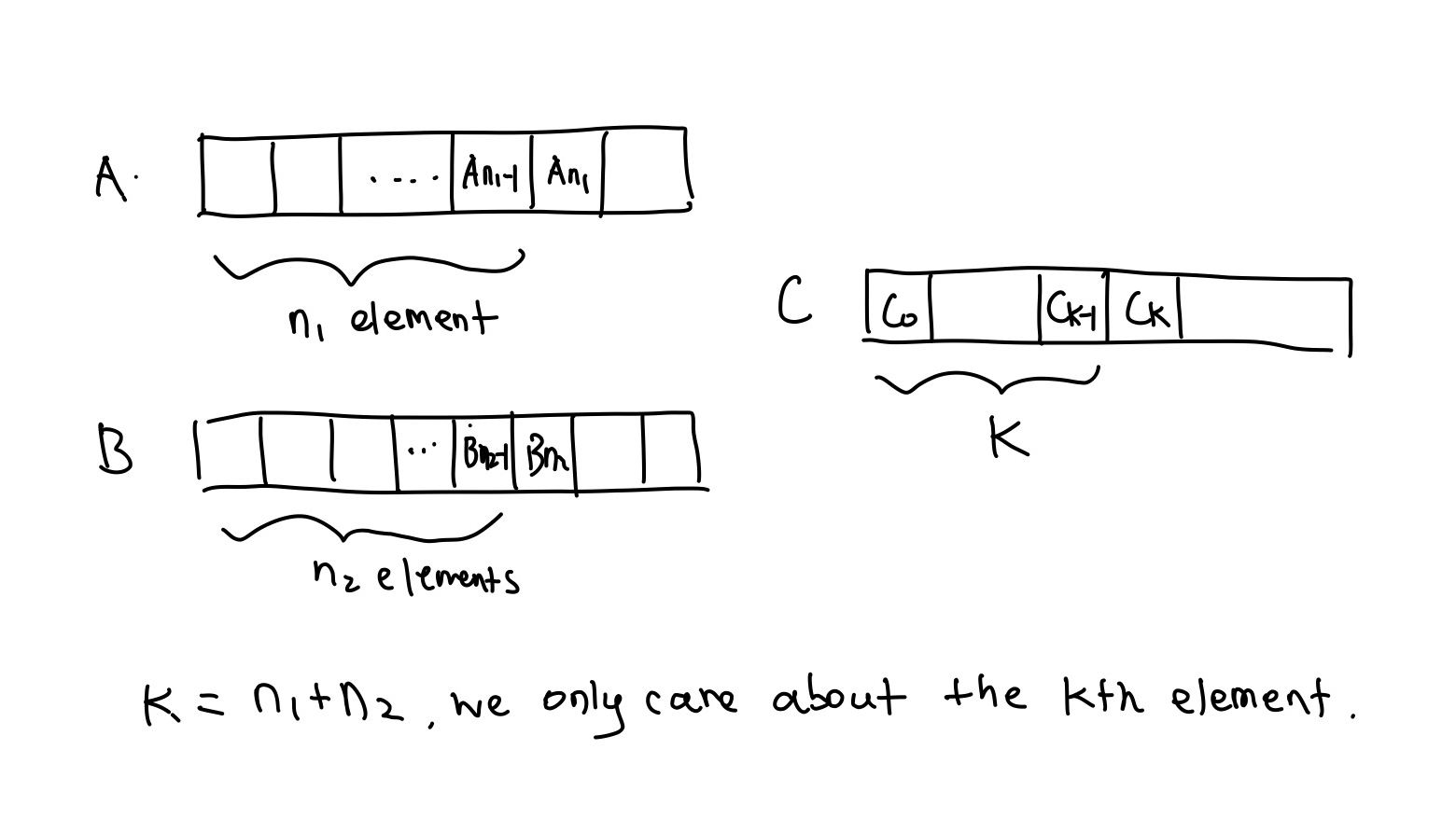# 两个有序数组的中位数self.__wrap_b=(t,n,e)=>{e=e||document.querySelector([data-br="\${t}"]);let s=e.parentElement,r=B=>e.style.maxWidth=B+"px";e.style.maxWidth="";let o=s.clientWidth,u=s.clientHeight,a=o/2-.25,c=o+.5,p;if(o){for(r(a),a=Math.max(e.scrollWidth,a);a+1<c;)p=Math.round((a+c)/2),r(p),s.clientHeight===u?c=p:a=p;r(c*n+o*(1-n))}e.__wrap_o||(typeof ResizeObserver!="undefined"?(e.__wrap_o=new ResizeObserver(()=>{self.__wrap_b(0,+e.dataset.brr,e)})).observe(s): false&&0)};self.__wrap_b(":R4pmmmja:",1)

### 有序

A，B merge 后的 array, C, 的中位数，也能把这个 array 分成两部分。以下均假设 C 的长度是偶数（如果是奇数，一半的元素数量是floor(n/2) + 1，这一点在代码中有处理到）。如[1 2 3 4 5 6]，那么它的一半的长度为 3。由于这个数组里的全部元素都来自 A 和 B，前半段里肯定有n1来自 A，n2来自 B。### 怎么求 n1 和 n2

随便切一刀
L1   R1
A = a0 a1 a2 | a3 ... am
L2   R2
B = b0 b1 b2 b3 | b4 ... bn
随便切一刀
L1   R1
A = a0 a1 a2 | a3 ... am
L2   R2
B = b0 b1 b2 b3 | b4 ... bn

### 如何排除元素

• $L_1 > R_2$

• $L_2 > R_1$

看看下一刀可以切哪里
?  ?  L1   R1
A = a0 a1 a2 | a3 ... am
L2   R2
B = b0 b1 b2 b3 | b4 ... bn
看看下一刀可以切哪里
?  ?  L1   R1
A = a0 a1 a2 | a3 ... am
L2   R2
B = b0 b1 b2 b3 | b4 ... bn

### 代码和几个细节

def findMedianSortedArrays(nums1, nums2):
n1 = len(nums1)
n2 = len(nums2)

# make sure we're searching in the shorter one
if n1 > n2: #
return self.findMedianSortedArrays(nums2, nums1)

# If there's nothing in nums1, just return median of nums2
if not n1: return (nums2[(n2 - 1) // 2] + nums2[(n2) // 2]) / 2

k = (n1 + n2 + 1) // 2

cutL, cutR = 0, n1 #
cut1 = 0

while (cutL <= cutR):
cut1 = cutL + (cutR - cutL) // 2
cut2 = k - cut1

L1 = nums1[cut1 - 1] if cut1 > 0 else float('-inf')
R1 = nums1[cut1] if cut1 < n1 else float('inf')
L2 = nums2[cut2 - 1] if cut2 > 0 else float('-inf')
R2 = nums2[cut2] if cut2 < n2 else float('inf')

if L1 > R2:  # need smaller L1, move left
cutR = cut1 - 1
# 
elif L2 > R1:  # need larger R1, move right
cutL = cut1 + 1
else:  # right cut
size = n1 + n2
if size % 2 == 0:  # even
return (max(L1, L2) + min(R1, R2)) / 2
else:
return max(L1, L2)
return -1
def findMedianSortedArrays(nums1, nums2):
n1 = len(nums1)
n2 = len(nums2)

# make sure we're searching in the shorter one
if n1 > n2: #
return self.findMedianSortedArrays(nums2, nums1)

# If there's nothing in nums1, just return median of nums2
if not n1: return (nums2[(n2 - 1) // 2] + nums2[(n2) // 2]) / 2

k = (n1 + n2 + 1) // 2

cutL, cutR = 0, n1 #
cut1 = 0

while (cutL <= cutR):
cut1 = cutL + (cutR - cutL) // 2
cut2 = k - cut1

L1 = nums1[cut1 - 1] if cut1 > 0 else float('-inf')
R1 = nums1[cut1] if cut1 < n1 else float('inf')
L2 = nums2[cut2 - 1] if cut2 > 0 else float('-inf')
R2 = nums2[cut2] if cut2 < n2 else float('inf')

if L1 > R2:  # need smaller L1, move left
cutR = cut1 - 1
# 
elif L2 > R1:  # need larger R1, move right
cutL = cut1 + 1
else:  # right cut
size = n1 + n2
if size % 2 == 0:  # even
return (max(L1, L2) + min(R1, R2)) / 2
else:
return max(L1, L2)
return -1
1. 为什么一定要在短的那个里搜索？

我们需要保证k-cut1是一个非负数，因为最少也就是有零个元素来自某个数组。

$k = (n_1 + n_2) / 2$。但如果我们不选择短的那个，cut1 就有可能大于 k。那为什么短的那个不可能出现cut1 > k?

我们限制了$cut_1 \leq n_1 \leq n_2$, 如果$cut_1 > k$，那么$n_1 + n_2 \gt 2k$。如果 C 的长度是偶数，k 正好是一半的元素，这个自相矛盾；如果是奇数，k 为一半的元素加一，比一半的元素还多，2k 更是超过了 C 的长度，矛盾。

2. cutR为什么可以是n1，不是超过数组长度了么？

首先要明确 cutR 代表了什么。cutL 和 cutR 代表了这个 cut 可能出现的区间。如果 cut 在 n1 这个位置，证明 A 里面的全部元素都在前半段，也就是 A 的 max 小于 B 的 min。这里我们在边界条件已经处理过了。

3. 这里的elif可以是if么？能不能再多排除一点？

假设这两个条件同时成立，$L_1\gt R_2, L_2 \gt R_1$，那么$R_1 \geq L_1 \gt R_2 \geq L_2 \Rightarrow R_1 \gt L_2$$L_2 \gt R_1$矛盾，所以是不可能的。

Find me on Twitter or write me an email